Mobile QR Code1. (Department of ECE and ISRC, Seoul National University, Seoul 08826, Korea)

Neuron device, spiking neural network, neuron circuit, neuromorphic systems

## I. INTRODUCTION

Deep neural network (DNN) algorithms, which are regarded as an artificial intelligence (AI) technology, have become a core technology in various areas such as autonomous vehicles, image processing, and speech recognition [1-5]. Moreover, the technology has improved human convenience in many applications, leading to the start of new and innovative industries and research based on the DNNs. This great success of DNNs has been achieved with the explosive growth of ‘big data’ and the development of a processor that can quickly process the big data . In particular, a graphics processing unit (GPU) that consists of highly parallel processing cores can accelerate the DNN operations, such as vector-by-matrix multiplications (VMMs) and multiply-accumulate (MAC) operations. Thus, GPUs allow the training process of DNNs to be performed with a large amount of training data and advanced training techniques that require a high computational cost, which significantly improves the performance of DNNs.

Despite the great success of the DNNs, the high-performance DNNs still require a large number of parameters to be trained. For example, K. He et al. reported Resnet-1001 consisting of more than 1000 layers , and J. Lee et al. reported BERT network with more than 110M parameters to be trained . In the conventional von Neumann computing architectures such as GPUs and central processing units (CPUs), the memory and the processor are physically separated from each other, and the communication between them is performed in serial. Thus, the architectures have been suffered from the increasing power consumption and system latency when a large amount of data is communicated between the separated memories and processors [9-12]. This ‘memory wall’ problem becomes more serious as the size of the communicated data increases. In this regard, hardware-based spiking neural networks (SNNs), which are inspired by biological nervous systems, have been actively studied to overcome such limitations of von Neumann computing systems [13-15]. The hardware-based SNNs store a large number of parameters into the internal memory devices that can also compute the given operations. The SNNs with memory devices can efficiently perform large-scale parallel operations according to Ohm's and Kirchhoff's laws, thus exponentially reducing power consumption compared to traditional computing systems. Besides, it has been reported that the neuronal behavior of SNNs is similar to the behavior of an activation function in DNNs . In other words, the training process of SNNs can be done in DNNs, and then the training results (i.e. weights) can be transferred to the SNNs. As such, the SNNs can achieve near the state-of-the-art performance of DNNs while consuming low power.

Hardware-based SNNs mainly consist of two components: a synapse and a neuron. In particular, the neuron plays a key role as a bio-plausible processor that converts synapse information into spike signals (spike rate or time). Thanks to the efficient converting behavior of neurons, the neurons can replace analog-to-digital and digital-to-analog converters (AD/DACs) that require a large area and substantial power consumption in conventional neural systems . In this regard, many studies have been reported to implement artificial neurons using complementary metal-oxide-semiconductor (CMOS) circuits with various functionalities. G. Indiveri reported a low-power integrate-and-fire (IF) neuron circuit with a self-adaptation leaky integration function for a homeostasis function . W.-M. Kang et al. also reported an IF neuron circuit suitable for hardware-based SNNs. Based on CMOS IF neuron circuit, it has been reported that the steep switching characteristics of electronic devices can further save the energy consumption in the spike generation . Various emerging electronic devices have been proposed as neuron devices, for example, a two-terminal resistive random access memory (RRAM) , a phase-change random access memory (PCRAM) , a magnetic random access memory (MRAM) , floating-body FET , and a ferroelectric based FET . Also, capacitor-less neuron device can reduce significantly the neuron area by replacing the membrane capacitor, which occupies a large footprint, as a device with the memory function . These advantages of the neuron devices make hardware-based SNNs more efficient and promising. However, while the interest in high-potential neuron devices has been exploding, a comprehensive study on the comparison between the neuron devices in various aspects is still insufficient.

In this work, recent trends of neuron devices to implement the efficient hardware-based SNNs are reviewed. Firstly, conventional CMOS neuron circuits that mimic the biological neuron’s behavior are reviewed. Various types of emerging electronic devices that can replace the devices in the neuron circuits are introduced to further improve the neuron circuits. We also discuss the advanced neuron circuits that consist of the emerging neuron devices and CMOS circuits.

## II. CMOS NEURON CIRCUITS

### 1. CMOS Neuron Circuits

Basic operations of neurons in hardware-based SNNs are as follows. When the spike signals are applied to the synapses, the currents flow through the synapses and are summed up along the post-synaptic neurons. The neuron integrates the synaptic currents into its membrane capacitor (an integration part), and generates an output spike when the membrane potential by the accumulated currents exceeds the threshold voltage of the neurons (a spike generation part). The generated spike resets the membrane potential to the resting potential (reset part). One of the basic CMOS neuron circuits is Axon-Hillock neuron circuit, as shown in Fig. 1 . The Axon-Hillock neuron circuit has two capacitors: membrane capacitor ($\textit{C}$$_{\mathrm{mem}}) for integration part and feedback capacitor (\textit{C}$$_{\mathrm{fb}}$). Also, the circuit has one amplifier (Amp) for the spike generation part, and one reset MOSFET (M$_{\mathrm{reset}}$) for the reset part. The amplifier is typically implemented with two inverters; the circuit consists of two capacitors and 5 MOSFETs. $\textit{I}$$_{\mathrm{exc}} and \textit{I}$$_{\mathrm{inh}}$ represent the excitatory and inhibitory currents, respectively. As the currents are integrated into the membrane capacitor, the membrane potential ($\textit{V}$$_{\mathrm{mem}}) changes. When the \textit{V}$$_{\mathrm{mem}}$ reaches the threshold voltage ($\textit{V}$$_{\mathrm{th}}) of the amplifier, the \textit{V}$$_{\mathrm{out}}$switches from 0 V to $\textit{V}$$_{\mathrm{dd}}. Then the \textit{V}$$_{\mathrm{mem}}$ is increased by the $\textit{C}$$_{\mathrm{fb}}. Also, the increased \textit{V}$$_{\mathrm{out}}$ turns on the reset MOSFET, and the reset current reduces the membrane potential to the resting potential.

Based on the Axon-Hillock neuron circuit, integrate-and-fire (IF) neuron circuits were proposed [18, 19, 27], which show better switching ability for the spike generation. Fig. 2(a) shows a conventional IF neuron circuit with a comparator for the spike generation part and feedback MOSFET (M$_{\mathrm{fb}}$) . The comparator can be a differential amplifier or 2 inverters connected in series. When the membrane potential modulated by the $\textit{I}$$_{\mathrm{exc}} and \textit{I}$$_{\mathrm{inh}}$ is over the $\textit{V}$$_{\mathrm{th}} in the comparator, the output voltage of the comparator swings up and the output signal of Inv1 swings down. Note that the output voltage of Inv1 turns on the M_{\mathrm{fb}}, and the current of M_{\mathrm{fb}} charges the membrane capacitor. The increased membrane potential by the current of M_{\mathrm{fb}} increases the output voltage of the comparator. Fig. 2(b) shows another CMOS IF neuron circuit . This circuit does not use the feedback MOSFET that is directly connected to the membrane capacitor, whereas the positive feedback also operates in the circuit. When the membrane potential exceeds the \textit{V}$$_{\mathrm{th}}$, the trigger device (M1) is turned on. Then, the voltage at node 1 decreases, and the output of Inv1 increases. The output of Inv1 turns off M4 and turns on M2. The voltage at node 1 decreases again, further increasing the output of Inv1. This positive feedback operation can boost the switching speed when a spike is generated. In addition, the pull-down output of Inv2 turns on M3 and turns off M5 after the discharging delay by the capacitor connected to the gate of M3. This delay determines the spike width at the output of the IF neuron circuit. Also, the membrane potential is decreased to the resting potential by M$_{\mathrm{reset}}$.

Leaky integrate-and-fire (LIF) neuron circuits mimicking the ion diffusion through the membrane were proposed . In the LIF neuron circuits, the membrane potential exponentially decays when the synaptic currents do not flow into the membrane capacitor, which means ‘leaky’. This behavior can be easily implemented with a resistor (leaky resistor, $\textit{R}$$_{\mathrm{L}}) connected between the membrane capacitor in the IF neuron circuits and the ground. The resistor constantly discharges the membrane capacitor, decreasing the membrane potential over time. If the \textit{R}$$_{\mathrm{L}}$ is an infinite value, the LIF neuron circuits become IF neuron circuits with zero leakage current.

##### Fig. 1. Axon-hillock circuit (a) Schematic diagram, (b) membrane potential and output voltage over time. Reproduced from. CC BY 4.0.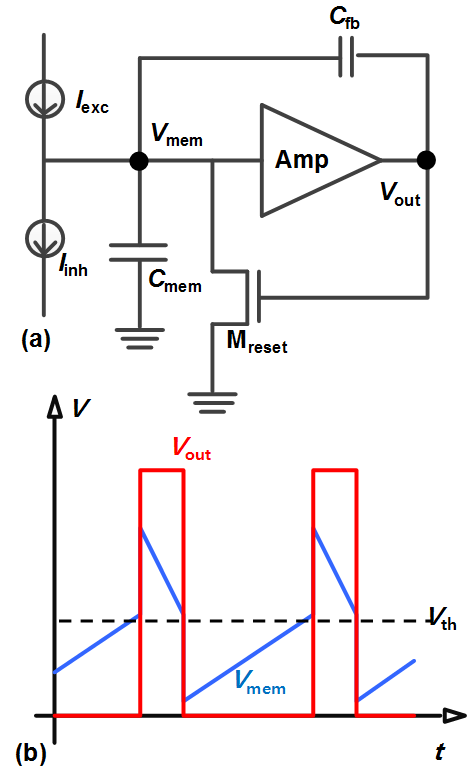##### Fig. 2. Integrate-and-fire (IF) neuron circuits proposed in (a), (b).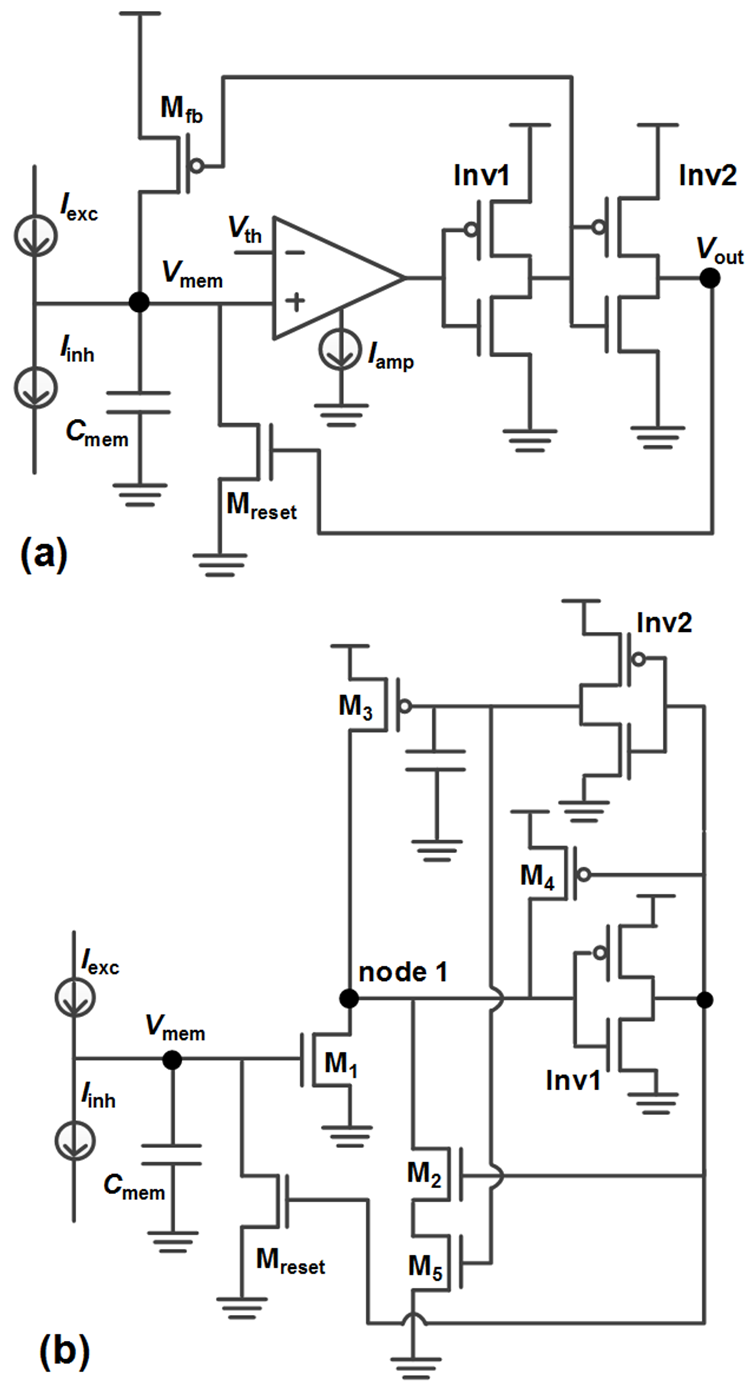### 2. Limitations of CMOS Neuron Circuits

Although the conventional CMOS neuron circuits can mimic the biological behavior of neurons successfully, the neuron circuits are still facing some limitations in large-scale hardware-based SNNs: energy consumption to generate a spike and a large-area membrane capacitor. Since the positive feedback can boost the switching speed of the spike generation part when the membrane potential exceeds $\textit{V}$$_{\mathrm{th}}, IF neuron circuits can save energy consumption. However, when the membrane potential increases to near \textit{V}$$_{\mathrm{th}}$of neurons (not exceeds), the static current of MOSFET also increases in the inverters, leading to large energy consumption in the spike generation. If the membrane potential near $\textit{V}$$_{\mathrm{th}} maintains for a long time, the energy consumption of the neuron becomes more significant. Fig. 3(a) shows a reported neuron circuit using a MOSFET as a trigger device . Here, when the membrane potential reaches the threshold voltage of the trigger MOSFET, the neuron generates a spike. Fig. 3(b) shows the static current of the trigger MOSFET in the conventional neuron circuit before the membrane potential reaches \textit{V}$$_{\mathrm{th}}$. The neuron circuit is set to generate a spike when 25 input pulses are applied to the membrane capacitor. The time the static current steeply decreases is when a spike is generated in the neuron. As shown in Fig. 3(b), the static current of the trigger MOSFET exponentially increases as the membrane potential increases. Therefore, the high static current can be an important topic to implement efficient hardware-based SNNs.

A large-area membrane capacitor is also an important issue to be discussed. Since the training capacity of neural networks increases as the network size increases, the number of neurons should also increase for high-performance SNNs. For example, in the VGG-16 network that has shown high performance in Imagenet classification, 24M neurons are required . If each neuron in the SNNs with the VGG-16 network structure has a large-area membrane capacitor, the total area of the membrane capacitors will be a significant problem. Furthermore, in a cross-point synapse array, the synaptic devices are densely arranged with a pitch of bit lines (BLs) and word lines (WLs). Then, the neuron circuit with a large-area membrane capacitor clearly exceeds the pitch of WLs and BLs, causing the synapses to share the neuron circuits . Then, the operations in SNNs with the shared neuron circuits should be performed sequentially, and the great advantage of parallelism in SNNs fades away.

As an approach to overcome the limitations of CMOS neuron circuits, novel neuron devices have attracted attention. The neuron devices replace the following components in the neuron circuits: 1) the trigger device in the spike generation part (shown in Fig. 3(a)), 2) the membrane capacitor in the integration part, and 3) both the trigger device and membrane capacitor. Neuron devices replacing the trigger device exhibit steeper swing than MOSFET device, and neuron device replacing the membrane capacitor changes their conductance states with smaller area than the capacitor. The advanced neuron circuits with the emerging neuron devices can save the energy consumption to generate a spike and the area of membrane capacitor, compared to the CMOS neuron circuits. A detailed discussion of the neuron devices will be described in the next sections.

##### Fig. 3. (a) Conventional neuron circuit whose trigger device is MOSFET. When the membrane potential turns on the trigger device, the neuron circuit generates a spike. The reset pulse is generated in the self-controller, (b) The static current of the trigger MOSFET over time. The neuron is designed to generate a spike when 25 input pulses are applied. Reproduced from. CC BY 4.0.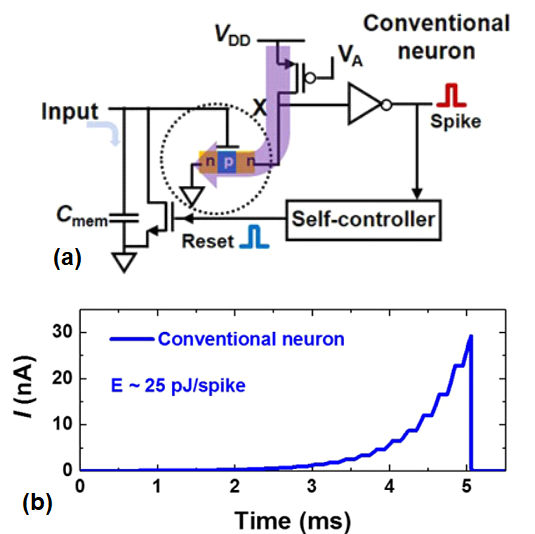## III. NEURON DEVICES

### 1.Resistive Random Access Memory

An RRAM is a 2-terminal non-volatile memory device based on the resistance switching . Many kinds of RRAMs have been reported as candidates for synaptic devices since they are easy to design a weight matrix using a cross-point RRAM array with long-term memory functionality. Fig. 4(a) shows the typical structure of 2-terminal RRAM devices with a metal-insulator-metal (MIM) structure. The RRAM device exhibits resistance switching characteristics that depend on the conditions (amplitude and pulse rate) of the applied set voltage, as shown in Fig. 4(b) and (c). In this regard, as input pulses from the synapse arrays are applied to RRAM devices that mimic neurons, the devices can accumulate the input signals in the resistance of the devices, mimicking the integration part of IF neurons. Because the SET and RESET processes in RRAM devices operate at low voltages, RRAM-based neuronal devices do not require peripheral circuitry to generate high voltages. The switching mechanisms of RRAMs depend on the type of insulating material, typically filamentary switching. The SET process of RRAMs is attributed to the dielectric soft breakdown and creation of conductive filaments, usually consisting of oxygen vacancies. The RESET process is attributed to the rupture of the conductive filaments, usually caused by recombination of oxygen vacancies with oxygen ions migrated from the electrode/oxide interfacial reservoir.

P. Stoliar et al. demonstrated that the LIF neuron model can be implemented by a single-component device based on a Mott insulator compound . The lacunar spinel compounds AM4Q8 (A = Ga, Ge; M = V, Nb, Ta, Mo; Q = S, Se) containing transition-metal tetrahedral clusters are narrow-gap Mott insulators with Mott–Hubbard gaps in the order of 0.1–0.3 eV. The system implements the LIF function similar to the biological neurons. It is worth noting that this novel functionality of the Mott device can go beyond the LIF model since it readily implements a spike by delivering an outgoing current pulse. Besides, C. Adda et al. proposed a neuron device with the LIF function by using narrow gap Mott insulators like the canonical system (V$_{\mathrm{1-x}}$Cr$_{\mathrm{x}}$)$_{2}$O$_{3}$ . The mechanism of electric Mott transition is the collapse of the Mott insulating state when voltage pulses (40 V, 20 $μ$s) are applied to the Mott insulator of (V$_{\mathrm{0.89}}$C$_{\mathrm{0.11}}$)$_{2}$O$_{3}$. Filament formation as the number of pulses for the integration function shows leaky integration of artificial neurons with various pulse intervals. J. Lin et al. proposed capacitor-less RRAM-based stochastic neuron [33,34]. The filament length in RRAM-based neurons can be represented as the membrane potential in the conventional CMOS neuron circuit. Applying a pulse (1.2 V, 10 $μ$s) to the RRAM can mimic the integration function by accumulating a charge in the membrane capacitor of a conventional neuron. By changing the resistance of RRAM, the fire function of neurons is implemented in the RRAM-based neurons. The reported energy consumption of RRAM in read operations is 2.14 pJ/spike at a pulse width of 50 ns. M.-W. Kwon et al. proposed Ag/Si$_{3}$N$_{4}$/TiN (MIM)-based RRAM to replace the membrane capacitor in the neuron circuit using SET process of RRAM . If the input pulse (2 V, 20 $μ$s) is biased continuously, the metal ions reach the bottom electrode (BE) and form a conducting metal bridge between the top electrode (TE) and BE. Then, the RRAM switches into LRS, and the current is increased abruptly from 10 $μ$A (threshold current) to 6 mA. By applying the negative bias to the TE, the conducting filaments are ruptured. Thus, the conductivity is decreased gradually and resets the RRAM-based neuron. The Pr$_{\mathrm{0.7}}$Ca$_{\mathrm{0.3}}$MnO$_{3}$ (PCMO) based RRAM has been researched for the synaptic devices due to its scalability with good endurance and retention . S. Lashkare et al. proposed emerging bipolar PCMO based RRAM as the neuron device with analog conductance change . The resistive switching mechanism in the PCMO is the transport of oxygen ions to the reactive electrode. The oxygen ions in the PCMO with positive voltage drift towards the electrode. By applying a negative voltage (SET pulse: -2.5 V, 1 $μ$s) to RRAM, oxygen ions drift from the reactive electrode and fill the vacancies. When the current at a read bias of PCMO-based neuron device is over 50 $μ$A (threshold current), the output spike is generated, which can mimic the IF neurons. In this regard, RRAM is an attractive device for mimicking biological neurons in terms of 2-terminal structure, low power, CMOS compatibility, and high-density scalability. However, additional circuits such as comparators or differential amplifiers are still required to compare the resistance of RRAM for the spike generation.

##### Fig. 4. (a) Schematic of RRAM used as the neuron device, (b) turn-on time of RRAM as a parameter of set voltage amplitude, (c) Spike rate of RRAM as the number of set pulses. © 2018 IEEE. Reprinted, with permission, from.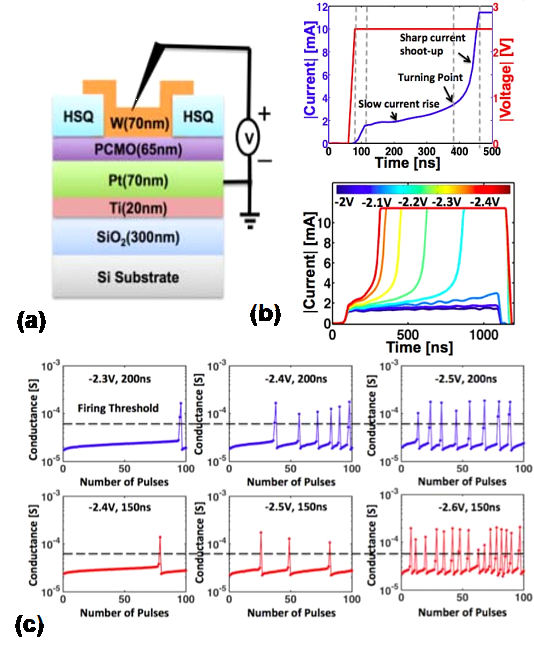### 2.Phase Change Memory

PCMs have been widely researched as synaptic devices due to their good memory characteristics such as retention (~10 years), endurance (>10$^{8}$), and fast set speed . Conventional PCMs consist of a top electrode, phase change material, heater, and bottom electrode. The resistance of PCM is determined by the phase change states of an inter-layer (phase change material) in PCM. A Ge$_{2}$Sb$_{2}$Te$_{5}$ (GST) has been widely used as the phase change material. The amorphous and crystalline phases of the GST have high resistance state (HRS) and low resistance state (LRS), respectively. To create an amorphous region within the crystalline material, a voltage pulse of sufficiently high amplitude is applied to PCM. Then, the current flows between the phase change material and heater, inducing the Joule heating. A substantial portion of the crystalline phase in PCM is melted like mushroom boundary by the Joule heating power. In PCM, the process of changing the phase change material from amorphous to crystalline phase is called SET. Likewise, the process of changing the phase change material into an amorphous phase is called RESET. Using the process of melting the substantial portion of the crystalline phase in PCM, C. D. Wright et al. and T. Tuma et al. proposed PCM-based neuron devices [38,39]. The SET process that applies crystallizing pulses (2 V, 20 ns) to PCM is represented with accumulating charges in the membrane capacitor for the integration function. After the SET process, changing LRS in PCM is represented to the fire function of neurons (threshold current: 2 $μ$A). Fig. 5 shows a schematic of PCM used as the neuron device and the conductance of the device as a function of the number of crystallizing pulses. C. D. Wright et al. and T. Tuma et al. demonstrated that PCM can be implemented as the function of IF neurons by the SET process in phase change materials.

Despite the great advantages of PCMs, Joule heating power that is required to crystallize the phase change material consumes substantial power per spike during the integration. Since PCM-based neuron devices have stochastic switching characteristics with the number of pulses, as shown in Fig. 5(b), the development of neural networks suitable for stochastic switching characteristics is necessary.

##### Fig. 5. (a) Phase-change device conductance as a parameter of the number of crystallizing pulses for the pulse sequence, (b) Spike rate of the PCM as a parameter of amplitude of the input pulses. © 2016 Nature Publishing Group. Reprinted, with permission, from.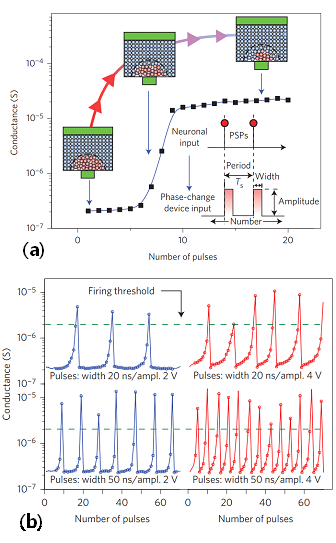### 3.Magnetic Random Access Memory

An MRAM has been researched as one of the candidates in emerging synaptic devices for hardware-based neural networks. As synaptic devices, MRAMs have relatively good performance in the read and write operations with the high speed (~ ns) and low energy consumption (~ pJ) . Also, MRAMs show excellent characteristics in terms of endurance (>10$^{8}$). MRAMs are generally categorized into spin-transfer torque (STT) and spin-orbit torque (SOT) MRAMs, depending on the spin mechanism. Fig. 6(a) and (b) show new structures of STT- and SOT-MRAMs to mimic the IF function, respectively. Conventional STT-MRAMs with a magnetic tunnel junction (MTJ) consist of a free magnetic layer, a tunnel layer, and a fixed magnetic layer [40-45]. When the magnetization direction of the free magnetic layer is parallel to the fixed magnetic layer, a resistance state of the STT-MRAM is low. On the other hand, the resistance state of the STT-MRAM is high when the direction of the free magnetic layer is anti-parallel to that of the fixed magnetic layer. Thus, the resistance state of STT-MRAM is determined by the magnetization direction of the free magnetic layer with respect to the fixed magnetic layer. Flowing the current through the MTJ can change the magnetization direction (spin-up or spin down) of the free magnetic layer due to the STT effect. The SOT-MRAM consists of a ferromagnetic layer and a heavy metal layer [46,47]. In the SOT-MRAM, the current flowing through the heavy metal causes SOT operation, changing the magnetization direction (spin up and spin down) of the magnetic layer. A resistance state of the SOT-MRAM is determined by moving a domain wall that is a boundary between spin-up and spin-down in the magnetic layer. In addition, when the same current flows to change the magnetization direction, the magnetization of the SOT mechanism is larger than that of the STT mechanism . Therefore, the SOT-MRAM has a lower power consumption during the write operation than the STT-MRAM. However, the SOT-MRAM has three or four terminals to allow current to flow through the heavy metal to change the resistance state. Conventional STT-MRAMs show the binary memory function by changing the magnetic direction in the free magnetic layer. To mimic the integration function of neurons, D. Fan et al. proposed an STT neuron device with 4-terminals based on domain wall motion (DWM) magnetic strip . By applying current pulses (~1 ns) in the lateral direction to the 4-terminal STT neuron device, the DWM of the STT neuron device moves in the lateral direction. When the conductance of the STT neuron device with the DWM is over the threshold current (~200 $μ$A) of the reference MTJ, the output signal is generated, and reset current is applied to the STT neuron device in a lateral direction to initialize displacement of the domain wall. To implement the multi-level resistance in the STT-MRAM with binary memory for synaptic and neuron devices, D. Zhang et al. proposed multiple vertically stacked MTJs . The MTJ consists of the CoFeB/MgO/CoFeB thin films and shows stable multiple resistance states by interfacial and materials engineering of its components. By changing the resistance of multiple MTJ stacks, the integrate-and-fire function of neurons is represented. K.~Kondo et al. and D. W. Kim et al. proposed a 2-terminal perpendicular STT based neuron device, which consists of the bottom electrode/seed layer/synthetic anti-ferromagnetic layer (SyAF)/ ferromagnetic free layer/MgO tunneling layer/ferromagnetic pinned layer/top electrode [42,43]. The proposed p-STT neuron devices have double free magnetic layers to implement the integration function of the membrane capacitor. When the voltage pulses (~1.2~V, 250 $μ$s) are applied to the p-STT neuron device, the spin-direction of electrons in the first-free layer near the MgO tunneling barrier tends to change from downward to upward due to the STT mechanism. When the voltage pulses (synaptic spikes) are continuously applied to the p-STT neuron device after changing the spin-direction of all electrons in the first-free layer completely, the spin-direction of all electrons in the second-free layer is changed from the parallel (10 k${\Omega}$) to the anti-parallel state (~23 k${\Omega}$) by the ferro coupling and STT mechanism. As a result, the resistance of p-STT neuron device rapidly increases. In the p-STT neuron device, the integrate-and-fire function of neurons was implemented by gradually changing the magnetic direction of the first free magnetic layer. M.-H. Wu et al. and F.-X. Liang et al. proposed a p-STT with dual-MgO/CoFeB interfaces and a Co/Pt multilayer SyAF-based pinned layer [44,45]. The proposed STT neuron device exploits a back-hopping (BH) oscillation mechanism in the MTJ, implements integration, voltage spike generation, and reset operation in a single device. To generate output spikes, synaptic current and extra bias current (0.8 mA) are needed. A. Kurenkov et al. proposed antiferromagnet/ferromagnet hetero-structure using the SOT mechanism for the neuron device . The SOT neuron device with 4-terminals consists of a stack of Ta/Pt/Pt$_{38}$Mn$_{62}$/Pt/(Co/Ni)$_{2}$/Co/MgO/Ru into 100 nm devices with binary switching. By applying voltage pulses (~1 V, 100 ns) through the heavy metal, the domain wall moves in the lateral direction due to the SOT mechanism. The integrate-and-fire function of neurons is implemented by changing the resistance of the SOT neuron device with the DWM. Although the SOT-based neuron device has low energy consumption to rotate spin-up and spin-down, development for high density is needed because of the 4-terminal structure of the heavy metal and the area to move the domain wall for the integration function.

##### Fig. 6. (a) Structure of STT-MRAM and the resistance change of STT-MRAM with number of pulses. Reproduced from. CC BY 4.0, (b) Characteristics of the SOT-MRAM with displacement of the domain wall. © 2019 WILEY-VCH. Reprinted, with permission, from.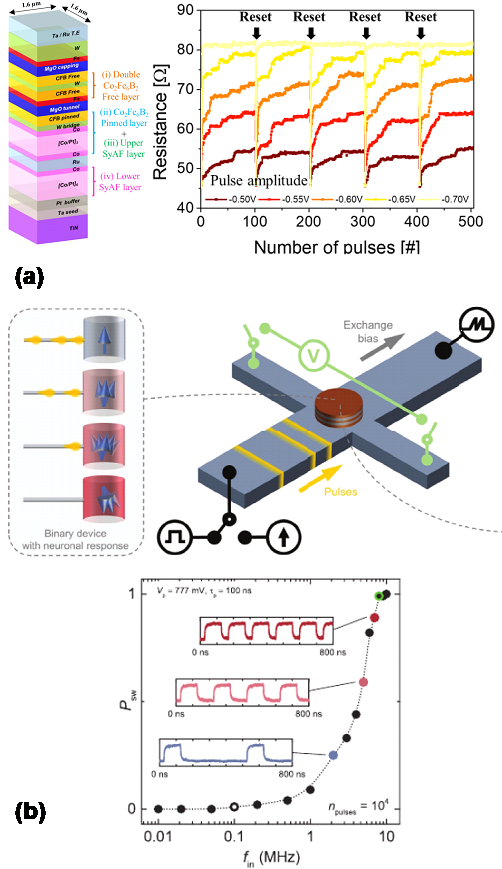### 4.Floating-body FET

Based on the mature Si technology that has been widely studied in several decades, various FET-type neuron devices were proposed [17, 23, 25, 47-49]. Thanks to their high compatibility with CMOS process technology, the studies on the FET-type neuron devices have been extended to the advanced neuron circuits that can overcome the limitations of conventional CMOS neuron circuits. One of the popular FET-type neuron devices is a positive feedback FET device. Fig. 7(a) shows a typical structure of a positive feedback FET device with a $\textit{pnpn}$-doped floating body and two gates . The detailed operation of the device is as follows. When the positive bias is applied to gate 1 and drain, the electrons are injected from the $\textit{n}$$^{+} source into the \textit{n}$$^{-}$ doped floating body. The electrons are accumulated in the floating body and lower the barrier height for the holes. Then, the holes are also injected from the $\textit{p}$$^{+} drain into the \textit{p}$$^{-}$ doped floating body, lowering the barrier height for the electrons. Thus, the electrons are more easily injected into the $\textit{n}$$^{-} body, lowering the barrier height for the holes again. This positive feedback operation flattens the energy band, which leads to the diode current flowing through the device. Fig. 7(b) shows the energy band of the device depending on the off-state and on-state. At the on-state, the energy band of the device is flat. Fig. 7(c) and (d) show the drain current versus \textit{V}$$_{\mathrm{gate1}}$ depending on the $\textit{V}$$_{\mathrm{drain}} and \textit{V}$$_{\mathrm{gate2}}$, respectively. Since the drain voltage determines the diode current when the positive feedback FET device is the on-state, the drain current exponentially increases as the drain voltage increases. In addition, since the depth of the potential well in the $\textit{n}$$^{-} doped floating-body increases with increased \textit{V}$$_{\mathrm{gate2}}$, the turn-on voltage of $\textit{V}$$_{\mathrm{gate1}} increases as \textit{V}$$_{\mathrm{gate2}}$ increases. Note that the most important characteristic of the positive feedback FET device is subthreshold swing (SS). Due to the positive feedback operation, the device shows super-steep switching characteristics when the $\textit{V}$$_{\mathrm{gate1}}is the turn-on voltage of the device. The reported SS is less than 2.3 mV/dec, which is much lower than the SS of MOSFETs. This strong advantage makes the positive feedback FET device replace the trigger MOSFET in the CMOS neuron circuits, reducing the energy consumption in the spike generation operation. Additionally, based on the positive feedback FET device, a novel concept of the neuron device that can replace a membrane capacitor was proposed . The floating body in the device acts as a capacitor that accumulates the injected electrons, which can be used for the membrane capacitor. As a result, the neuron device can replace not only the trigger MOSFET with the super-steep switching characteristics but also the membrane capacitor that significantly occupies a large area. Fig. 8(a) shows a schematic view of the neuron device based on the positive feedback FET. In this neuron device, the synaptic current is converted into the voltage signal, and the voltage signal is applied to the G1 of the positive feedback FET. The positive bias applied to G1 increases the amount of electrons in the \textit{n}-doped floating body. When the gate bias exceeds the turn-on voltage of the positive feedback operation, the device steeply turns on (reported SS = 0.015 mV/dec). On the contrary, the positive bias applied to G2 decreases the amount of electrons in the \textit{n}-doped floating body, which inhibits the energy band from flattening out. In this behavior of the positive feedback FET, the input signals (excitatory and inhibitory signals) are accumulated into the floating body, which replaces the membrane capacitor. Thus, the device can greatly reduce the footprint of hardware-based SNNs. In addition, thanks to the separated gates receiving each signal, the device simultaneously processes the excitatory and inhibitory signals. By using both excitatory and inhibitory signals, S. Y. Woo et al. reported a 98.58% of accuracy rate for MNIST classification in the SNNs. Another approach to using floating-body FETs as neuron devices is to use the single transistor latch (STL) phenomenon in 3-terminal floating body FETs (Fig. 9) . In floating body FETs with negative gate bias, it is observed that the drain current is dramatically increased at a drain bias of \textit{V}$$_{\mathrm{latch}}$, as shown in Fig. 9(b). This means that the resistance between the drain and source also steeply changes at the $\textit{V}$$_{\mathrm{latch}}, and the steep switching behavior of the 3-terminal floating body FET can be utilized as neuron devices replacing the trigger MOSFET in the CMOS neuron circuit. Also, the body-drain junction capacitor in the floating body FET can be used to replace the membrane capacitor, which reduces the area of the membrane capacitor in SNNs. As a result, the behavior of LIF neurons was implemented with the STL phenomenon in the 3-terminal floating body FETs while saving the energy consumption to generate a spike. The mentioned floating body FETs have shown promising potential to replace the trigger MOSFET and membrane capacitor in CMOS neuron circuits. On the other hand, there are some limitations in using the FETs as neuron devices. Floating body FETs using positive feedback operations have a time delay to switch their states at the turn-on voltage (~100 ns, ). Thus, the time interval between the spikes in SNNs should be much longer than the time delay, which can increase the system latency of SNNs. The biggest limitation of floating body FETs is the variation between the devices, such as the turn-on voltage of positive feedback operations. The variation in turn-on voltage directly affects the \textit{V}$$_{\mathrm{th}}$ of the spike generation part, degrading the accuracy of SNNs. To solve the variation problem, a charge trapping layer (CTL) can be employed in the gate insulator stack of the FETs. Since the charge in the CTL modulates the electric field, the turn-on voltage of the devices can be adjusted to the set voltage. Besides, an incremental step pulse programming (ISPP) method more accurately adjusts the turn-on voltage of positive feedback FETs with the CTL. Using the tuning methods, the turn-on voltages of the neuron devices can be uniform on the chip.

##### Fig. 7. (a) Schematic of the positive feedback FET, (b) Energy band of the positive feedback FET depending on the on-state and off-state. Drain current versus gate 1 voltage as a parameter of (c) drain voltage, (d) gate 2 voltage. © 2018 AIP Publishing. Reprinted, with permission, from.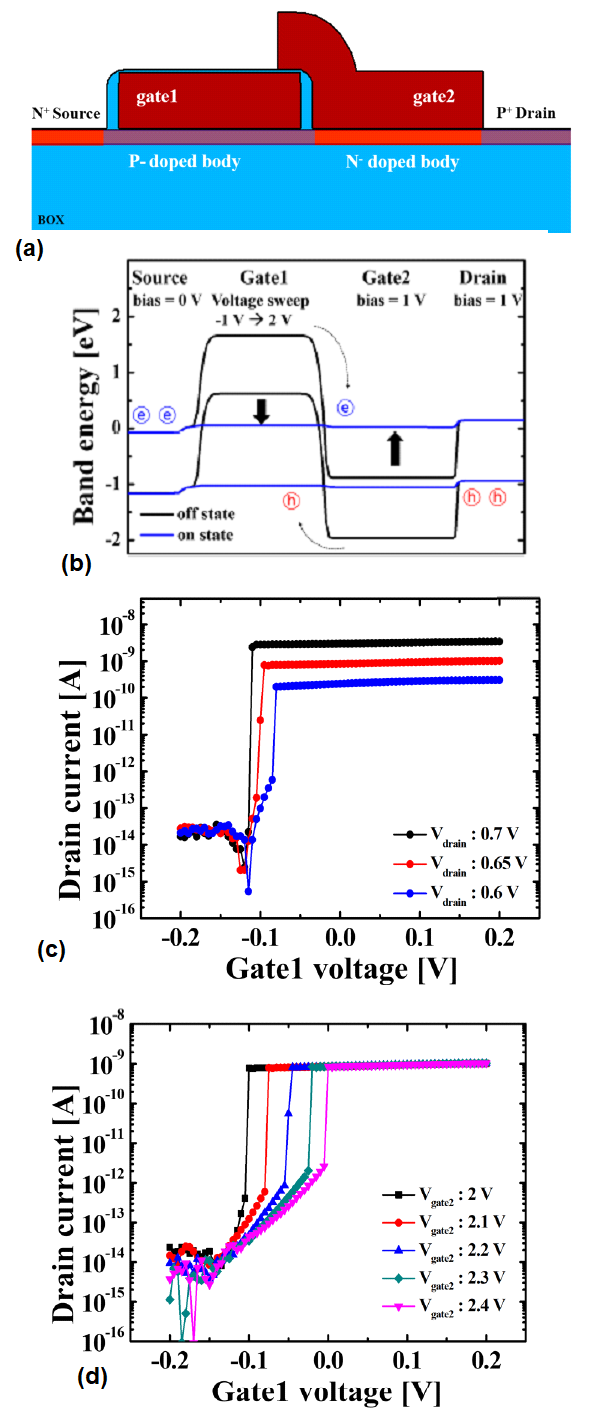##### Fig. 8. (a) Schematic of the positive feedback FET, (b) SEM image of the fabricated positive feedback FET, (c) Anode current versus V$_{\mathrm{G1}}$as a parameter of V$_{\mathrm{G2}}$, (d) Drain current versus V$_{\mathrm{G1}}$ as a parameter of V$_{\mathrm{G2}}$. Reproduced from. CC BY 4.0.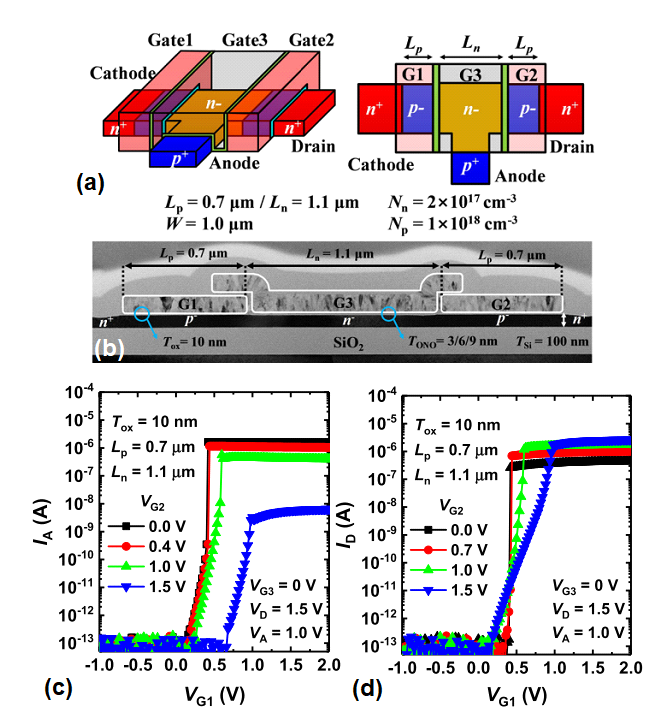##### Fig. 9. (a) Schematic of floating body FET on an SOI wafer, (b) Drain current versus drain voltage as a parameter of V$_{\mathrm{GS}}$. Steep switching is shown by the single transistor latch (STL) phenomenon. © 2020 IEEE. Reprinted, with permission, from.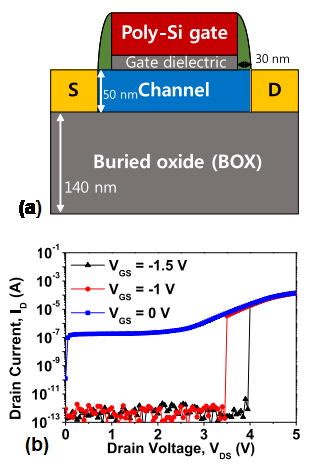### 5.Ferroelectric FETs

Recently, ferroelectric FETs (FeFETs) whose gate stack consists of a ferroelectric layer such as Hf$_{\mathrm{x}}$Zr$_{\mathrm{1-x}}$O$_{2}$material have been actively researched for neuron devices [50-53]. In the ferroelectric layer, the polarization can be accumulated with the strength and applied time of the electric field according to the multi-domain switching theory . In other words, the ferroelectric polarization can be modulated with the voltage pulses (synaptic inputs) applied to the FeFET. Note that the accumulated ferroelectric polarization also affects the conductance of the FeFETs. Thus, the FeFET can switch when the threshold voltage of FeFETs crosses the read voltage caused by the voltage pulses. This behavior of the ferroelectric layer in FeFETs can replace the membrane capacitor of conventional CMOS neuron circuits, which can reduce the area footprint of hardware-based SNNs. The big advantage of the FeFET neuron devices is that the voltage amplitude modulating the accumulated polarization is low compared to the voltage amplitude in FETs with the CTL. In the neuron device with the CTL , the input voltage pulses that modulate the conductance of the neuron device are over ~7 V. Hence, the operating voltage of the system should be increased, which is a burden of the system. In contrast, the ferroelectric polarization can be modulated with the ~3 V of voltage pulses, reducing the operating voltage of the system while taking the advantages of the FeFET neuron devices.

Fig. 10(a) shows the typical structure of metal-ferroelectric-metal (MFM) capacitor with Hf$_{\mathrm{x}}$Zr$_{\mathrm{1-x}}$O$_{2}$ material. The 10 nm Hf$_{\mathrm{x}}$Zr$_{\mathrm{1-x}}$O$_{2}$is sandwiched between the Tungsten electrodes. Fig. 10(b) shows the polarization versus electric field loop with minor loops by multiple domains in the ferroelectric material. By using the characteristics of a ferroelectric layer, an FeFET-based LIF neuron was proposed . Fig. 11(a) shows the schematic structure of FeFET with a leaky ferroelectric layer. The ferroelectric layer shows a leaky effect through degradation in polarization. Fig. 11(b)-(d) shows the drain current of FeFET versus pulse number as parameters of pulse amplitude, pulse width, and the time interval between the pulses, respectively. The polarization accumulation increases with the increasing pulse amplitude and pulse width, but decreases with the increasing time interval due to the leaky effect. The conductance of FeFET neuron devices can be modulated by the polarization accumulation. In this regard, the input signals (voltage pulses) from the synapses can be applied to the gate of FeFET, and the ferroelectric layer can be accumulated the input signals. Thus, the FeFET can be used for a neuron device with 1T1R structure, which is much smaller in area than conventional CMOS neuron circuits with a large-area membrane capacitor.

Since the reported FeFET has a single gate that receives the input signals, the FeFET can process the excitatory signals only, which means that the direction in conductance change of FeFET is only the direction to generate a spike. However, the inhibitory signals are the essential elements for high-performance SNNs, thus the single-gate FeFETs as neuron devices are limited. In , J. Luo et al. also proposed a leaky-FeFET neuron that can process both excitatory and inhibitory signals by adding a transistor connected to the gate of FeFET. However, in this case, the excitatory and inhibitory signals should be processed sequentially because the single gate that processes the signals is used for a membrane capacitor. In terms of device structure or neuron circuit design, the FeFET should be further improved to process both excitatory and inhibitory signals simultaneously. In addition, SS of the FeFET devices is similar to the conventional MOSFET. Thus, the FeFET neuron devices are also facing the energy consumption in the spike generation operation.

The overall comparison of the reported neuron devices is represented in Table 1. The output voltage amplitude and number of transistors are also represented in Table 1.

##### Fig. 10. (a) Schematic of a ferroelectric layer with a Hf$_{\mathrm{x}}$Zr$_{\mathrm{1-x}}$O$_{2}$ material, (b) Polarization versus electric field with multi-domain. Reproduced from. CC BY 4.0.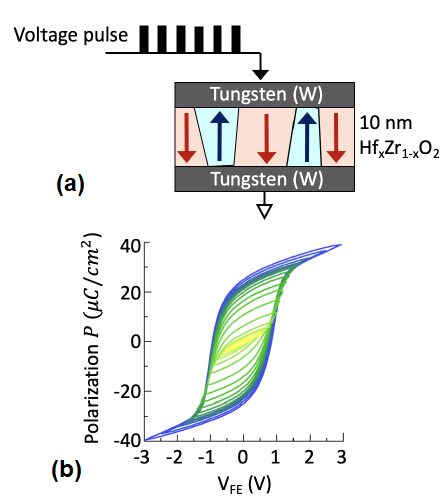##### Fig. 11. (a) Schematic of the L-FeFET, (b) Current accumulation effect of the L-FeFET depending on (a) pulse voltage, (b) pulse width, (c) time delay between the pulses. The accumulation of L-FeFET acts as a membrane capacitor that integrates the signals of synapses. © 2019 IEEE. Reprinted, with permission, from.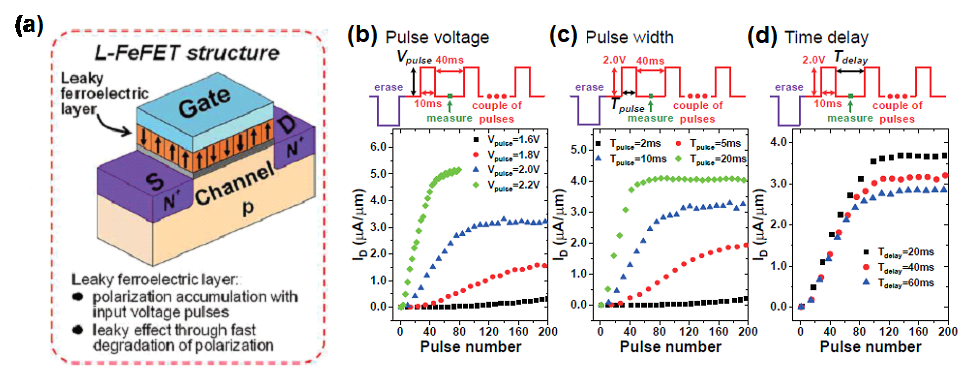##### Table 1. Comparison of reported neuron circuit and devices
 Type Material Mechanism Replacing Component Operation Voltage Threshold Current Output Voltage Amplitude Energy Consumption Number of Transistors in Neuron Circuit CMOS Circuit  Si - - 1.5 V 30 nA 1.5 V 25 pJ/spike 5 RRAM  W/PCMO/Pt Transport of Oxygen Ions Membrane Cap. 2.5 V > 10 μA - - - RRAM  Ag/HfO$_{2}$/Pt Formation of Filaments Trigger Device < 1.0 V ~ 1 pA 0.2 V 270 fJ/spike - PCM  Ge$_{2}$Sb$_{2}$Te$_{5}$ Joule Heating Membrane Cap. 2.0 V 2 μA - - > 9 SOT-MRAM  Ta/Pt/Pt$_{38}$Mn$_{62}$/ Pt/Co/Ni/Co/ MgO/Ru Magnetization Switching Membrane Cap. > 5.0 V - - - - Floating-Body FET  Si Positive Feedback Trigger Device 1.5 V < 10 pA 1.5 V 120 fJ/spike 9 Floating-Body FET  Si Positive Feedback Trigger Device, Membrane Cap. 1.0 V ~1 nA 1.0 V 0.62 pJ/spike 6 Floating-Body FET  Si Single Transistor Latch Trigger Device, Membrane Cap. ~3.0 V ~1 pA ~3.0 V 45 pJ/spike - FeFET  HZO, Si Polarization Switching Membrane Cap. 2.2 V 5 μA > 1.5 V - 4

In this section, we will discuss the advanced neuron circuits that include an emerging neuron device replacing a membrane capacitor or switching MOSFET. Fig. 12 shows an IF neuron circuit based on a p-STT MTJ replacing the membrane capacitor . In this circuit, the input pulses from the synapse array are applied to the MTJ. Then, the resistance of MTJ is increased by the input pulses, meaning that the input signals are accumulated into the MTJ. When the resistance of the MTJ exceeds the reference resistance, the IF neuron circuit generates a spike and resets the resistance of the MTJ. The calculated p-STT MTJ-based IF neuron’s area is ~8.2 $μ$m$^{2}$, which is greatly reduced by the area of a CMOS neuron circuit using a membrane capacitor. Fig. 13 shows an LIF neuron circuit with 2T1R structure based on an FeFET device . The voltage pulses from the excitatory synapses are applied to the gate of FeFET through the R0, the conductance of FeFET increases (decreasing $\textit{V}$$_{\mathrm{T}} of FeFET). In contrast, the voltage pulses from the inhibitory synapses are applied to the gate of nFET whose source is biased to \textit{V}$$_{\mathrm{inh}}$, decreasing the conductance of the FeFET (increasing $\textit{V}$$_{\mathrm{T}}$ of FeFET). Then, the input signals from the synapses are accumulated into the FeFET, replacing the membrane capacitor. When the V$_{\mathrm{T}}$ of the FeFET is lower than the read voltage, the high drain current flows through the FeFET, generating a spike signal.

Fig. 14 shows an IF neuron circuit based on positive feedback FET device that shows steep switching characteristics . In this circuit, the input signals are integrated into the membrane capacitor. When the N$_{\mathrm{pass}}$ turns on, the membrane potential is applied to the INV1. When the membrane potential exceeds the turn-on voltage of the neuron device, the conductance of the device increases sharply, and the voltage at node 1 decreases. The P$_{\mathrm{boost}}$ also turns on and charges the membrane capacitor. A spike is generated at the output node, and the reset nMOS turns on (N$_{\mathrm{discharge}}$). If the resistance of R1 is not infinite, this circuit becomes the LIF neuron circuit. Note that the energy consumption of the advanced neuron circuit is reduced by 94% than the conventional CMOS neuron circuit. Thanks to the steep switching characteristics of the positive feedback FET, the current through the inverter in the advanced neuron circuit is kept low when the membrane potential approaches close to the turn-on voltage of FET. The advanced neuron circuit significantly reduces the energy consumption of the neuron circuit. Although the large-area membrane capacitor is used in the advanced neuron circuit, this low energy consumption is a clear advantage.

The advanced neuron circuit whose neuron device replaces both membrane capacitor and switching device is shown in Fig. 15. Here, the neuron device is based on the positive feedback FET in . The excitatory and inhibitory signals are applied to gate 1 and gate 2, respectively, modulating the amount of accumulated electrons in the $\textit{n}$-body under gate 3. The turn-on voltage of the FET can be adjusted by the CTL under gate 3, allowing the turn-on voltage of the neuron devices to be uniform on the chip. When the excitatory signals are not applied to gate 1, the pMOS (M2) turns on and pulls up the input voltage of the inverter. When the accumulated electrons in the $\textit{n}$-body are enough to trigger the positive feedback due to the excitatory signals, the $\textit{pn}$ diode turns on and the input voltage of the inverter decreases. Then, a spike signal is generated at the output of the inverter, and the spike turns on nMOS (M1). The turn-on M1 pulls down the input voltage of the inverter, and then the accumulated electrons in the $\textit{n}$-body flow out to the cathode for the reset of the FET device. In this advanced neuron circuit, the positive feedback FET device replaces both membrane capacitor and switching device. The input signals from the synapse array (excitatory and inhibitory signals) are accumulated into the $\textit{n}$-body as a membrane capacitor, and the FET steeply switches on when the positive feedback operation is triggered by the accumulated electrons. The reported neuron circuit only consumes 0.62 pJ/spike, which is reduced by ~90% compared to that of the conventional CMOS neuron circuit.

##### Fig. 12. Advanced neuron circuit based on the MTJ. The input signals are accumulated as the resistance of MTJ, and the resistance is compared with the reference resistance. Reproduced from. CC BY 4.0.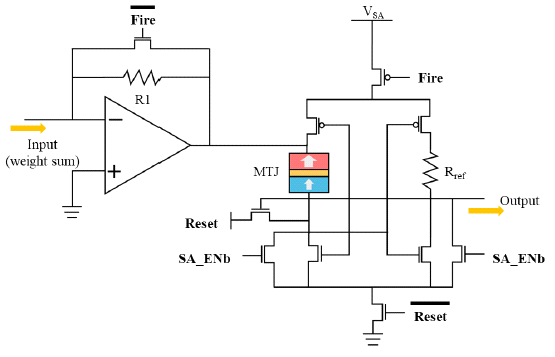##### Fig. 13. Advanced neuron circuit using a FeFET. The excitatory signals are applied through R0, and the inhibitory signals are applied to the gate of nFET. The entire neuron circuit was fabricated. © 2019 IEEE. Reprinted, with permission, from.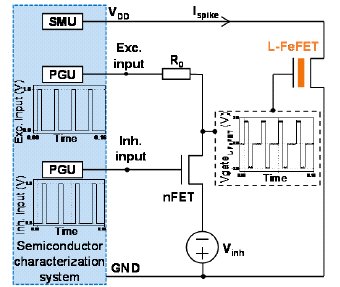##### Fig. 14. Advanced neuron circuit using a positive feedback FET. The FET replaces the trigger MOSFET in the conventional CMOS neuron circuit to reduce the energy consumption for spike generation. The entire neuron circuit was fabricated. © 2018 AIP Publishing. Reprinted, with permission, from.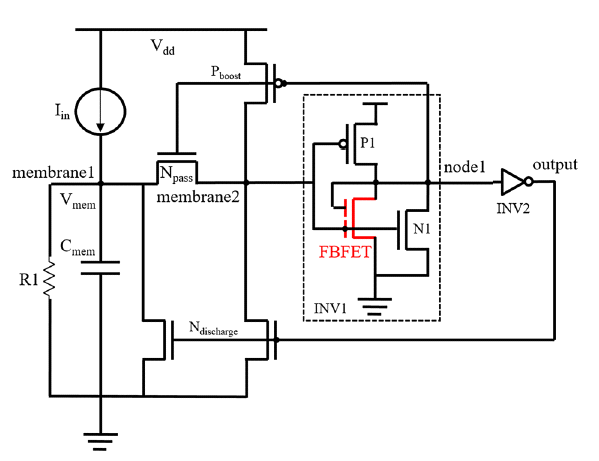##### Fig. 15. Advanced neuron circuit using a positive feedback FET. The excitatory and inhibitory signals are applied to the G1 and G2, respectively, and the signals are accumulated as the electrons in the floating n-body. The FET replaces a membrane capacitor and trigger device in the conventional neuron circuits. Reproduced from. CC BY 4.0.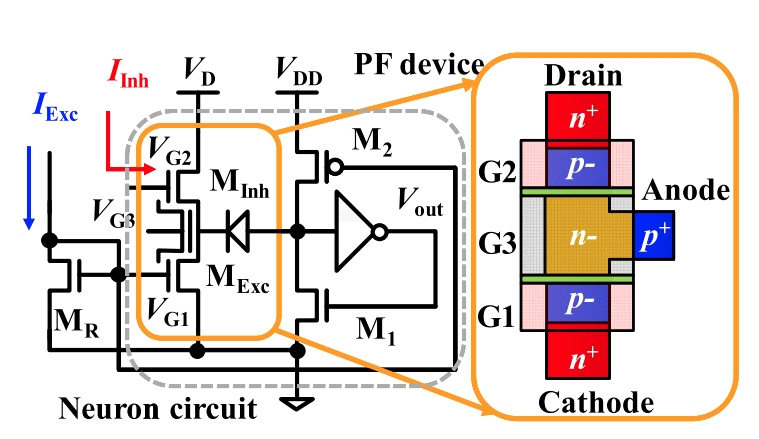## V. CONCLUSION

In this work, we reviewed recent trends of analog neuron devices for hardware-based SNNs. Firstly, conventional CMOS neuron circuits that mimic the biological behaviors of neurons were reviewed. The CMOS neuron circuits face limitations in that they consume relatively high energy consumption in the spike generation and the membrane capacitor that occupies a large area. In this regard, the emerging neuron devices were introduced to overcome such limitations of CMOS neuron circuits. We summarized the recent progress of RRAMs, PCMs, MRAMs, floating body FETs, and FeFETs as neuron devices. The reported neuron devices can replace the trigger device with the steep switching characteristics, and/or the membrane capacitor with their memory functionality. Finally, the advanced neuron circuits that include the emerging neuron devices were discussed.

### ACKNOWLEDGMENTS

This work was supported by the BK21 FOUR program of the Education and Research Program for Future ICT Pioneers, Seoul National University in 2022 and the National Research Foundation of Korea (NRF-2021R1A2C3009069).

### References

1
Hinton G. E., Salakhutdinov R. R., 2006, Reducing the dimensionality of data with neural networks, Science, Vol. 313, pp. 504-5072
Srivastava N., Hinton G. E., Krizhevsky A., Sutskever I., Salakhutdinov R., 2014, Dropout: a simple way to prevent neural networks from overfitting, J. Mach. Learn. Res., Vol. 15, pp. 1929-1958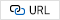3
Szegedy C., Liu W., Jia Y., Sermanet P., Reed S., An-guelov D., Erhan D., Vanhoucke V., Rabinovich A., 2015, Going deeper with convolutions, Proc. of the IEEE Conf. on Computer Vision and Pattern Recognition (CVPR)4
Gokmen T., Vlasov Y., 2016, Acceleration of deep neural network training with resistive cross-point devices: design considerations, Front. Neurosci., Vol. 10, pp. 3335
Kwon D., Lim S., Bae J.-H., Lee S.-T., Kim H., Seo Y.-T., Oh S., Kim J., Yeom K., Park B.-G., Lee J.-H., 2020, On-Chip Training Spiking Neural Networks Using Approximated Backpropagation With Analog Synaptic Devices, Front. Neurosci, Vol. 14, pp. 4236
Jeong D. S., Kim K. M., Kim S., Choi B. J., Hwang C. S., 2016, Memristors for Energy-Efficient New Computing Paradigms, Advanced Electronic Materials, Vol. 2, No. 9, pp. 16000907
He K., Zhang X., Ren S., Sun J., 2016, Deep residual learning for image recognition, Proc. of the IEEE Conf. on Computer Vision and Pattern Recognition (CVPR)8
Delvin J., Chang M.-W., Lee K., Toutanova K., 2019, BERT: Pre-training of Deep Bidirectional Trans-formers for Language Understanding, Arxiv9
Yu S., 2018, Neuro-inspired computing with emerging nonvolatile memorys, Proc. of the IEEE, Vol. 106, No. 2, pp. 260-28510
Kwon D., Jung G., Shin W., Jeong Y., Hong S., Oh S., Bae J.-H., Park B.-G., Lee J.-H., 2020, Low-Power and reliable Gas Sensing System Based on Recurrent Neural Networks, Sensors and Actuators B: Chemical, pp. 12925811
Woo J., Kim J. H., Im J.-P., Moon S. E., 2020, Recent Advancements in Emerging Neuromor-phic Device Technologies, Advanced Intelligent Systems, Vol. 2, pp. 200011112
Kim C.-H., Lim S., Woo S. Y., Kang W.-M., Seo Y.-T., Lee S.-T., Lee S., Kwon D., Oh S., Noh Y., Kim H., Kim J., Bae J.-H., Lee J.-H., p. , Emerging memory technologies for neuromorphic computing, Nanotechnology, Vol. 30, pp. 03200113
Hwang S., Chang J., Oh M.-H., Lee J.-H., Park B.-G., 2020, Impact of the Sub-Resting Membrane Potential on Accurate Inference in Spiking Neural Networks, Scientific Reports, Vol. 10, pp. 351514
Dutta S., Schafer C., Gomez J., Ni K., Joshi S., Datta S., 2020, Supervised Learning in All FeFET-Based Spiking Neural Networks: Opportunities and Chal-lenges, Front. Neurosci., Vol. 14, pp. 63415
O’Connor P., Neil D., Liu S. C., Delbruck T., Pfeiffer M., 2013, Real-time classification and sensor fu-sion with a spiking deep belief network, Front. Neurosci., Vol. 7, pp. 17816
Rueckauer B., Lungu I.-A., Hu Y., Pfeiffer M., Liu S.-C., 2017, Conversion of Continuous-Valued Deep Networks to Efficient Event-Driven Networks for Image Classification, Front. Neurosci., Vol. 11, pp. 68217
Sung C.-L., Lue H.-T., Wei M.-L., Ho S.-Y., Hu H.-W., Du P.-Y., Chen W.-C., Lo C., Yeh T.-H., Wang K.-C., Lu C.-Y., 2020, A Novel Super-Steep Slope (~0.015mV/dec) Gate-Controlled Thyristor (GCT) Func-tional Memory Device to Support the Integrate-and-Fire Circuit for Spiking Neural Networks, IEEE In-ternational Electron Devices Meeting (IEDM)18
Indiveri G., 2003, A low-power adaptive inte-grate-and-fire neuron circuit, Proceedings of the 2003 International Symposium on Circuits and Sys-tems (ISCAS)19
Kang W.-M., Kim C.-H., Lee S., Woo S. Y., Bae J.-H., Park B.-G., Lee J.-H., 2019, A Spiking neural network with a global self-controller for unsupervised learning based on spike-timing-dependent plasticity using flash memory synaptic devices, In 2019 International Joint Conference on Neural Networks (IJCNN)20
Chua L., 2011, Resistance switching memo-ries are memristors, Appl. Phys. A., Vol. 102, pp. 765-78321
Burr G. W., et al. , 2016, Recent progress in phase-change memory technology, IEEE J. Emer. Sel. Topics Circuits Syst., Vol. 6, No. 2, pp. 146-16222
Sengupta A., et al. , 2016, Hybrid Spintron-ic-CMOS Spiking Neural Network with On-Chip Learning: Devices, Circuits, Systems, Phys. Rev. Applied, Vol. 6, No. 6, pp. 06400323
Kwon M.-W., Baek M.-H., Hwang S., Park K., Jang T., Kim T., Lee J., Cho S., Park B.-G., 2018, Integrate-and-fire neuron circuit using positive feedback field effect transistor for low power operation, Journal of Applied Physics, Vol. 124, pp. 15190324
Chen C., Yang M., Liu S., Liu T., Zhu K., Zhao Y., Wang H., Huang Q., Huang R., 2019, Bio-Inspired Neurons Based on Novel Leaky-FeFET with Ultra-Low Hardware Cost and Advanced Functionality for All-Ferroelectric Neural Network, 2019 Symposium on VLSI Technology, pp. T136-T13725
Woo S. Y., Kwon D., Choi N., Kang W.-M., Seo Y.-T., Park M.-K., Bae J.-H., Park B.-G., Lee J.-H., 2020, Low-Power and High-Density Neuron Device for Simultaneous Processing of Excitatory and Inhibitory Signals in Neuromorphic Systems, IEEE Access, Vol. 8, pp. 202639-20264726
Indiveri G., et al. , 2011, Neuromorphic silicon neuron circuits, Front. Neurosci., Vol. 5, No. 7327
van Schaik A., 2001, Building blocks for elec-tronic spiking neural networks, Neural Networks, Vol. 14, pp. 617-62828
Indiveri G., Stefanini F., Chicca E., 2010, Spike-based learning with a generalized integrate and fire silicon neuron, Proceedings of the 2010 International Symposium on Circuits and Systems (ISCAS)29
Choi K.-B., Woo S. Y., Kang W.-M., Lee S., Kim C.-H., Bae J.-H., Lim S., Lee J.-H., 2018, A Split-Gate Positive Feedback Device With an Integrate-and-Fire Capability for a High-Density Low-Power Neuron Circuit, Front. Neurosci., Vol. 12, pp. 70430
Simonyan K., Zisserman A., 2015, Very Deep Convolutional Networks For Large-Scale Image Recognition, Arxiv31
Stoliar et al. P., 2017, A Leaky-Integrate-and-Fire Neuron Analog Realized with a Mott Insulator, Adv. Func. Mater., Vol. 27, pp. 160474032
Adda C., 2017, First demonstration of "Leaky Integrate and Fire" artificial neuron behavior on (V$_{\mathrm{0.95}}$Cr$_{\mathrm{0.05}}$)$_{2}$O$_{3}$ thin film, MRS Communications, Vol. 8, pp. 835-84133
Lin J., Yuan J., 2017, Capacitor-less RRAM-based stochastic neuron for event-based unsu-pervised learning, IEEE Bio. Cir. Sys. Conference (BioCAS), pp. 1-434
Lin J., Yuan J., 2018, Analysis and Simulation of Capacitor-Less ReRAM-Based Stochastic Neurons for the in-Memory Spiking Neural Network, IEEE Trans. Bio. Cir. Sys., Vol. 12., No. 5, pp. 1004-101735
Kwon M.-W., et al. , 2017, Integrate-and-Fire (I&F) Neuron Circuit Using Resistive-Switching Random Access Memory (RRAM), Journal of Nanosci-ence and Nanotechnology, Vol. 17, No. 5, pp. 3038-304136
Panwar N., et al. , 2015, Effect of mor-phological change on unipolar and bipolar switching characteristics in Pr$_{\mathrm{0.7}}$Ca$_{\mathrm{0.3}}$MnO$_{3}$ based RRAM, in Proc. Mater. Res. Soc. Symp., Vol. 1729, pp. 47-5237
Lashkare S., et al , 2018, PCMO RRAM for Integrate-and-Fire Neuron in Spiking Neural Networks, IEEE Elec. Dev. Lett., Vol. 39, No. 4, pp. 484-48738
Tuma T., et al. , 2016, Stochastic phase-change neurons, Nature nanotechnology, Vol. 11, pp. 693-69939
Wright C. D., et al. , 2012, Beyond von-Neumann Computing with Nanoscale Phase-Change Memory Devices, Adv. Func. Mater., Vol. 23, pp. 2248-225440
Fan D., et al. , 2015, STT-SNN: A Spin-Transfer-Torque Based Soft-Limiting Non-Linear Neu-ron for Low-Power Artificial Neural Networks, IEEE Trans. Nano., Vol. 14, No. 6, pp. 1013-102341
Zhang D., et al. , 2016, All Spin Artificial Neural Networks Based on Compound Spintronic Syn-apse and Neuron, IEEE Trans. on Bio. Cir. Sys., Vol. 10, No. 4, pp. 828-83642
Kondo K., 2018, A two-terminal perpen-dicular spin-transfer torque based artificial neuron, J. Phys. D: Appl. Phys., Vol. 51, pp. 50400243
Kim D. W., et al. , 2020, Double MgO-Based Perpendicular Magnetic Tunnel Junction for Artificial Neuron, Front. Neurosci., Vol. 30, pp. 1-944
Wu M.-H., et al. , 2019, Extremely Compact Integrate-and-Fire STT-MRAM Neuron: A Pathway to-ward All-Spin Artificial Deep Neural Network, Symposium on VLSI Technology, pp. T3-445
Liang F.-X., et al. , 2019, Stochastic STT-MRAM Spiking Neuron Circuit, International Symposium on VLSI Technology, Systems and Applications (VLSI-TSA), pp. 151-15246
Kurenkov A., et al. , 2019, Artificial Neuron and Synapse Realized in an Antiferromagnet/Ferromagnet Heterostructure Using Dynamics of Spin-Orbit Torque Switching, Adv. Mater., Vol. 31, No. 23, pp. 190063647
Kwon M.-W., Park K., Baek M.-H., Lee J., Park B.-G., 2019, A Low-Energy High-Density Ca-pacitor-Less I&F Neuron Circuit Using Feedback FET Co-Integrated With CMOS, IEEE Journal of Electron Devices Society, Vol. 7, pp. 1080-108448
Han J.-K., Seo M., Yu J.-M., Suh Y.-J., Choi Y.-K., 2020, A Single Transistor Neuron With Independently Accessed Double-Gate for Excitatory-Inhibitory Function and Tunable Firing Threshold Voltage, IEEE Electron Device Letters, Vol. 41, No. 8, pp. 1157-116049
Han J.-K., Seo M., Kim W.-K., Kim M.-S., Kim S.-Y., Kim M.-S., Yun G.-J., Lee G.-B., Yu J.-M., 2020, Mimicry of Excitatory and In-hibitory Artificial Neuron With Leaky Integrate-and-Fire Function by a Single MOSFET, IEEE Electron Device Letters, Vol. 41, No. 2, pp. 208-21150
Saha A. K., Ni K., Dutta S., Datta S., Gupta S., 2019, Phase field modeling of domain dynamics and polarization accumulation in ferroelectric HZO, Applied Physics Letters, Vol. 114, pp. 20290351
Fang Y., Gomez J., Wang Z., Datta S., Khan S. I., Raychowdhury A., 2019, Neuro-Mimetic Dy-namics of a Ferroelectric FET-Based Spiking Neuron, IEEE Electron Device Letters, Vol. 40, No. 7, pp. 1213-121652
Ni K., et al. , 2018, In-memory computing primitive for sensor data fusion in 28 nm HKMG FeFET technology, 2018 IEEE International Electron Devices Meeting (IEDM)53
Luo J., Yu L., Liu T., Yang M., Fu Z., Liang Z., Chen L., Chen C., Liu S., Wu S., Huang Q., Huang R., 2019, Capacitor-less Stochastic Leaky-FeFET Neuron of Excitatory and Inhibitory Connections for SNN with Reduced Hardware Cost, 2019 IEEE International Electron Devices Meeting (IEDM)54
Lee D., Kwak M., Moon K., Choi W., Park J., Yoo J., Song J., Lim S., Sung C., Banerjee W., Hwang H., 2019, Various Threshold Switching Devices for Integrate and Fire Neuron Applications, Advanced Electronic Materials, Vol. 5, pp. 1800866## 저자소개

Dongseok Kwon received the B.S. degree in electrical engineering from Pohang University of Science and Technology (POSTECH), Pohang, South Korea, in 2017. He is currently pursuing the M.S. degree with the Department of Electrical and Computer Engineering, Seoul National University (SNU), Seoul, South Korea. His current research interests include neuromorphic system and its application in computing.

Sung Yun Woo received the B.S. degree in electrical engineering from Kyungpook National University, in 2014. He is currently pursuing the Ph.D. degree with the Department of Electrical and Computer Engineering, Seoul National University (SNU), Seoul, Korea. He is with the Inter-University Semiconductor Research Center, SNU. His current research interests include neuromorphic systems and neural networks.

Jong-Ho Lee (F’16) received the Ph.D. degree from Seoul National University (SNU), Seoul, in 1993, in electronic engineering. He was a Post-Doctoral Fellow with the Massachusetts Institute of Tech-nology, Cambridge, MA, USA, from 1998 to 1999. He has been a Professor with the School of Electrical and Computer Engineering, SNU, since 2009.